International
Tables for
Crystallography
Volume D
Physical properties of crystals
Edited by A. Authier

International Tables for Crystallography (2006). Vol. D, ch. 2.4, pp. 330-331

## Section 2.4.4. Brillouin scattering in crystals

R. Vachera* and E. Courtensa

aLaboratoire des Verres, Université Montpellier 2, Case 069, Place Eugène Bataillon, 34095 Montpellier CEDEX, France
Correspondence e-mail:  rene.vacher@ldv.univ-montp2.fr

### 2.4.4. Brillouin scattering in crystals

| top | pdf |

#### 2.4.4.1. Kinematics

| top | pdf |

Brillouin scattering occurs when an incident photon at frequency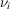interacts with the crystal to either produce or absorb an acoustic phonon at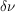, while a scattered photon at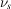is simultaneously emitted. Conservation of energy gives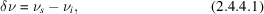where positivecorresponds to the anti-Stokes process. Conservation of momentum can be written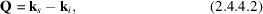where Q is the wavevector of the emitted phonon, and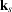,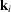are those of the scattered and incident photons, respectively. One can define unit vectors q in the direction of the wavevectors k by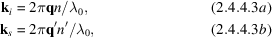where n and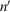are the appropriate refractive indices, andis the vacuum wavelength of the radiation. Equation (2.4.4.3b)assumes that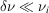so thatis not appreciably changed in the scattering. The incident and scattered waves have unit polarization vectorsand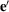, respectively, and corresponding indices n and. The polarization vectors are the principal directions of vibration derived from the sections of the ellipsoid of indices by planes perpendicular toand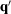, respectively. We assume that the electric vector of the light field Eopt is parallel to the displacement Dopt. This is exactly true for many cases listed in the tables below. In the other cases (such as skew directions in the orthorhombic group) this assumes that the birefringence is sufficiently small for the effect of the angle between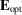and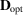to be negligible. A full treatment, including this effect, has been given by Nelson et al. (1972).

After substituting (2.4.4.3)in (2.4.4.2), the unit vector in the direction of the phonon wavevector is given by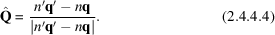The Brillouin shiftis related to the phonon velocity V by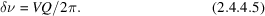Since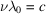, from (2.4.4.5)and (2.4.4.3), (2.4.4.4)one finds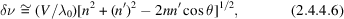whereis the angle betweenand.

#### 2.4.4.2. Scattering cross section

| top | pdf |

The power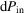, scattered from the illuminated volume V in a solid angle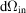, where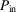and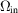are measured inside the sample, is given by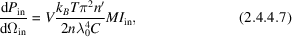where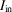is the incident light intensity inside the material,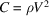is the appropriate elastic constant for the observed phonon, and the factor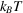results from taking the fluctuation–dissipation theorem in the classical limit for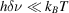(Hayes & Loudon, 1978). The coupling coefficient M is given by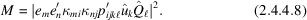In practice, the incident intensity is defined outside the scattering volume,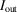, and for normal incidence one can write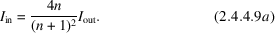Similarly, the scattered power is observed outside as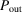, and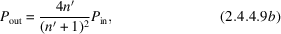again for normal incidence. Finally, the approximative relation between the scattering solid angle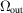, outside the sample, and the solid angle, in the sample, is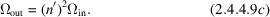Substituting (2.4.4.9a,b,c)in (2.4.4.7), one obtains (Vacher & Boyer, 1972)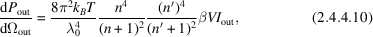where the coupling coefficientis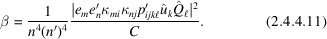In the cases of interest here, the tensoris diagonal,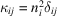without summation on i, and (2.4.4.11)can be written in the simpler form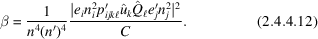### References

Hayes, W. & Loudon, R. (1978). Scattering of light by crystals. New York: Wiley.
Nelson, D. F., Lazay, P. D. & Lax, M. (1972). Brillouin scattering in anisotropic media: calcite. Phys. Rev. B, 6, 3109–3120.
Vacher, R. & Boyer, L. (1972). Brillouin scattering: a tool for the measurement of elastic and photoelastic constants. Phys. Rev. B, 6, 639–673.